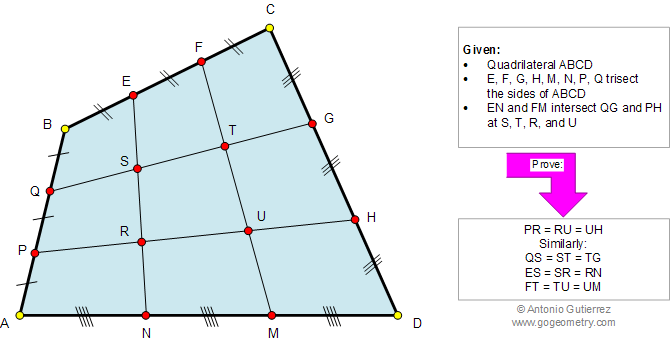# Geometry Problem 960. Quadrilateral, Trisection, Sides, Congruence, Similarity, Triangle

< PREVIOUS PROBLEM  |  NEXT PROBLEM >

 The figure below shows a quadrilateral ABCD. Points E, F, G, H, M, N, P, and Q trisect the sides (divide each side into three equal parts). Prove that EN and FM trisect QG and PH, respectively, similarly PH and QG trisect EN and FM.